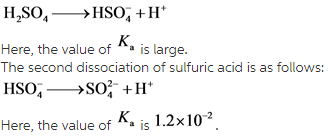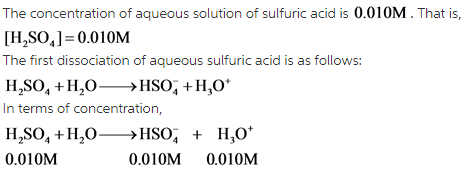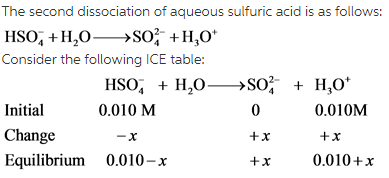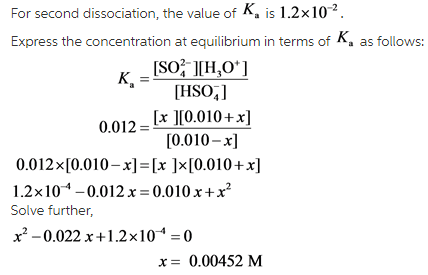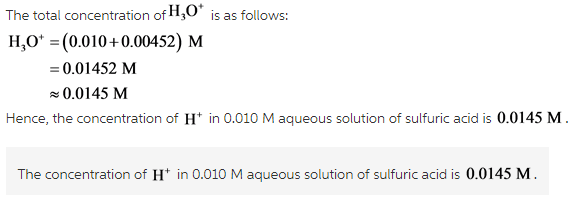# Calculate the concentration of H+ ions in a 0.010 M aqueous solution of sulfuric acid

Calculate the concentration of H+ ions in a 0.010 M aqueous solution of sulfuric acid.
Express your answer to three decimal places and include the appropriate units.
H+= [value] [units]

Concepts and reason
Sulfuric acid is a diprotic acid. A diprotic acid can be defined as an acid which can donate two Hydrogen atoms per molecule in an aqueous solution. Therefore, sulfuric acid donates its first proton to form hydrogen sulfate and the second one to form sulfate ion. However, it is to be noted that the loss of both the protons does occur at the same time. Each of the two dissociations takes place in a separate reaction as the strength of the acid is different at different times in a reaction because of the varying number of hydrogens attached to the molecule.
The dissociation constant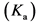provides information about the strength of an acid in a solution. For diprotic acids, there are twovalues which are different from each other. The first dissociation of sulfuric acid is complete while the second dissociation is not.

Fundamentals
The first dissociation of sulfuric acid is as follows: